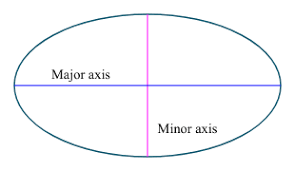## How to Calculate and Solve for the Axis and Area of an Ellipse | Nickzom CalculatorThe image above is an ellipse.

To compute the area of an ellipse, two essential parameters are needed and this parameters are axis (a) and axis (b).

A = πab

Where;
A = Area of the ellipse
a = Axis of the ellipse
b = Axis of the ellipse

Let’s solve an example;
Find the area of an ellipse when it has an axis (a) of 10 cm and an axis (b) of 17 cm.

This implies that;
a = Axis of the ellipse = 10 cm
b = Axis of the ellipse = 17 cm

A = πab
A = 3.142 x 10 x 17
A = 534.14

Therefore, the area of the ellipse is 534.14 cm2.

Calculating the Axis (a) of an ellipse using Area of the ellipse and Axis (b) of the ellipse.

a = A / πb

Where;
a = Axis of the ellipse
A = Area of the ellipse
b = Axis of the ellipse

Let’s solve an example;
Find the axis (a) of an ellipse when the area of the ellipse is 210 cm2 with an axis (b) of 19 cm.

This implies that;
A = Area of the ellipse = 210 cm2
b = Axis of the ellipse = 19 cm

a = A / πb
a = 210 / 3.142 x 19
a = 210 / 59.70
a = 3.52

Therefore, the axis (a) of an ellipse is 3.52 cm.